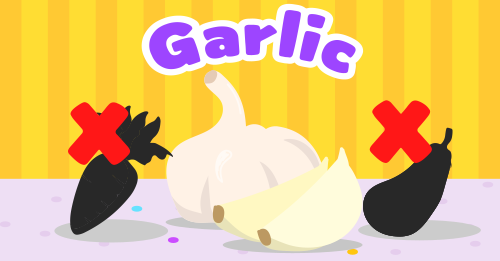Can you count how many cups of milk are there?Do you know the color of these airplanes?Let's count these peaches together!Can you find the correct capital letter?Which vegetable is bigger?Which one is the animal that we want?Can you tell the color of these buses?Do you know these numbers?Let's count hippos together!Can you tell the order of these animals?Let's count ice creams and sandwiches together!Can you count these compactors?Learn colors with trucks.Can you count these vihecles?Let's do some simple calculations.Can you count these strawberries and apples?Can you tell the first letters of these words of foods?Let's do some simple calculations.Can you tell the order of these animals?Can you count these cupcakes?